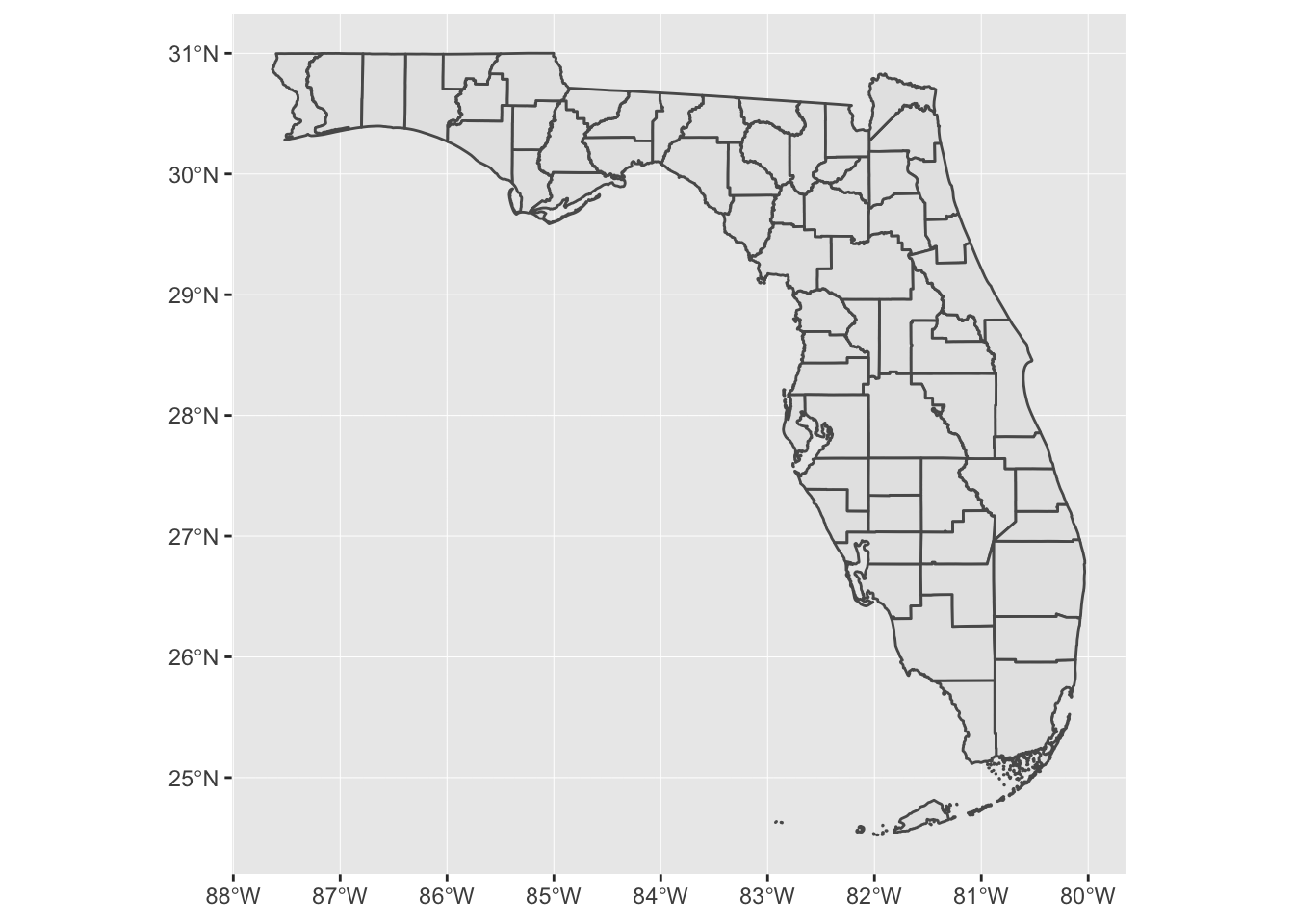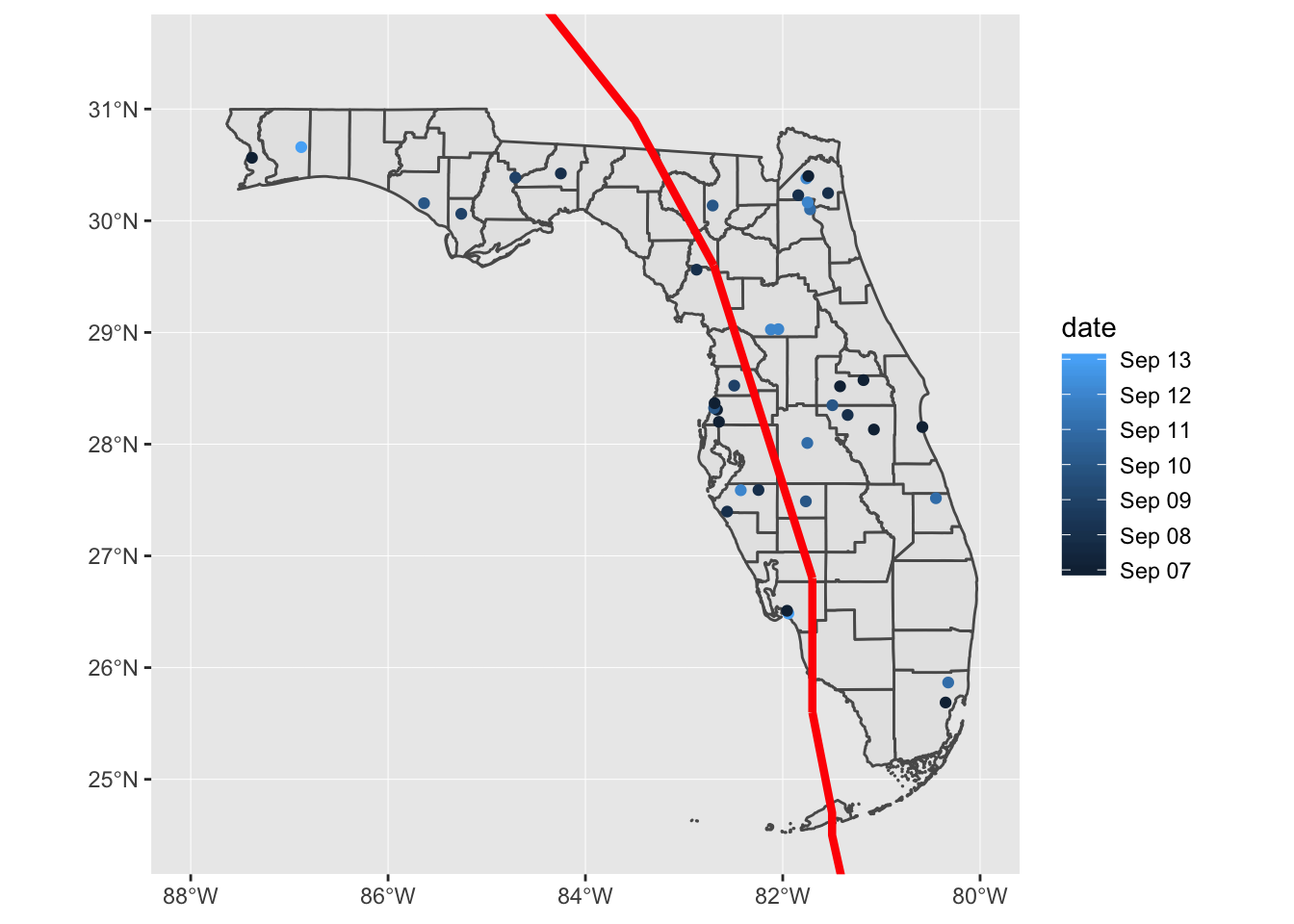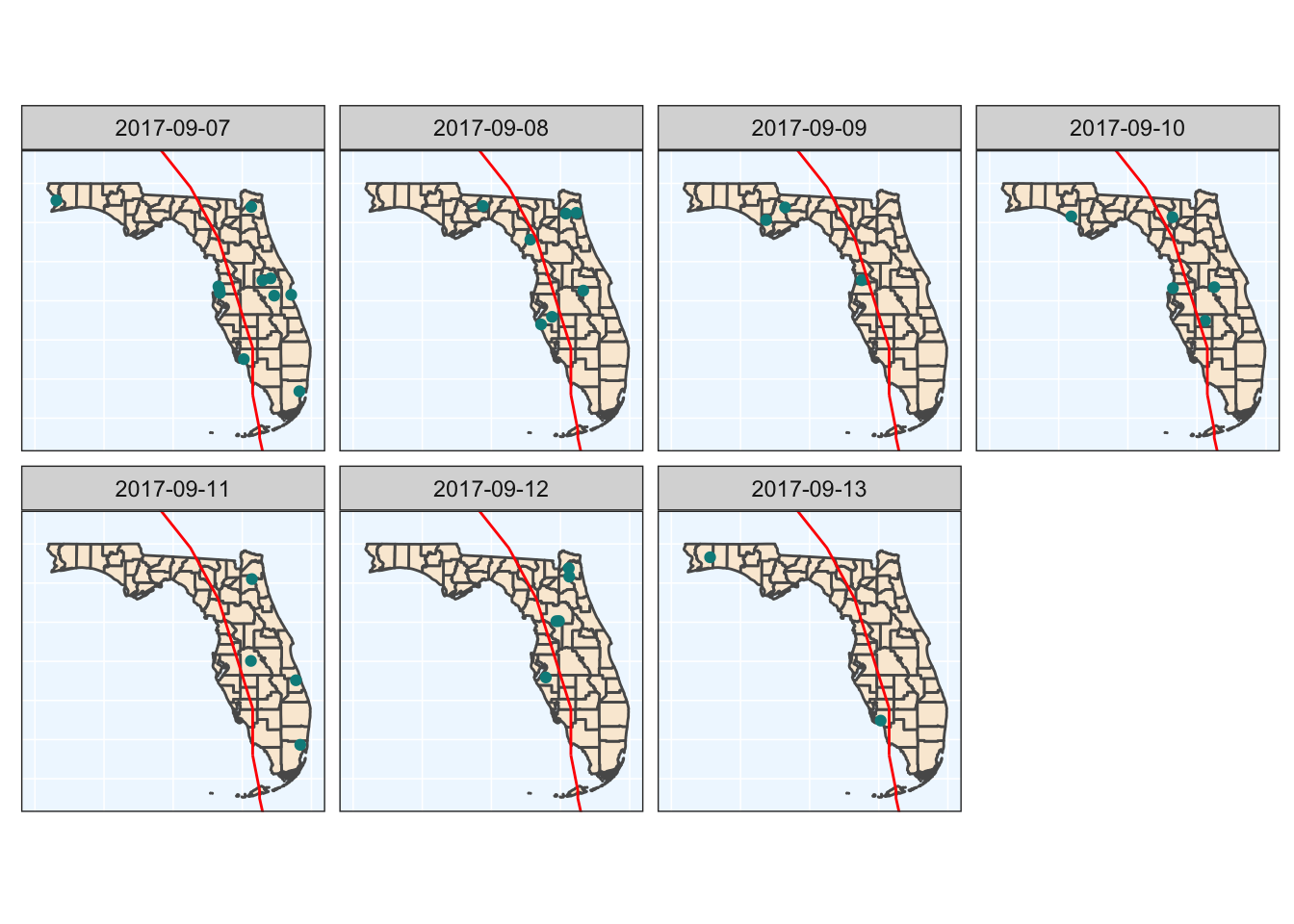## 3.2 Basic mapping

If you don’t have `ggplot2` still loaded from the exercises in the “Plot” section, you’ll need to load it. Then, you can create a `ggplot` object and add an `sf` layer to it with `geom_sf`, specifying the `sf` data set to plot.24 If you are running this on a Mac, there’s a chance that you might get an error message about “polygon edge not found.” There seems to be a bug that’s still being resolved involving the default graphics driver on Macs with this package. If you get this error message, try fixing it by adding the following layer to the `ggplot` code: `+ theme(axis.text = element_blank(), axis.ticks = element_blank()` Add a coordinate layer (`coord_sf`) appropriate for a map (otherwise, you might end up with a map that’s “stretched” out in either the x- or y-direction):

``````library("ggplot2")

ggplot() +
geom_sf(data = fl_counties) +
coord_sf()``````You can add other `sf` layers to this map, to add points for the location of each fatal accident and a line for the track of Hurricane Irma. Since the track for Irma began much further south than Florida and continues north of Florida, you’ll need to set `xlim` and `ylim` in the `coord_sf` call to ensure that the map is zoomed to show Florida. You can map aesthetics to values in the data, or to constant values, just like with a regular `ggplot` object. In this example, the color of each point is mapped to its date, while the track of Irma is shown in red and a bit larger than the default size.

``````ggplot() +
geom_sf(data = fl_counties) +
geom_sf(data = fl_accidents, aes(color = date)) +
geom_sf(data = irma_tracks, color = "red", size = 1.5) +
coord_sf(xlim = c(-88, -80), ylim = c(24.5, 31.5)) ``````You can do anything to this map that you would to a regular `ggplot` object. For example, instead of using color to show the date of an accident, you could use faceting to add a small map of each date using `facet_wrap`. This example code also adds some elements for the plot’s theme, including changing the background color and taking out the axis ticks and labels.

``````ggplot() +
geom_sf(data = fl_counties, fill = "antiquewhite") +
geom_sf(data = fl_accidents, color = "darkcyan") +
geom_sf(data = irma_tracks, color = "red") +
coord_sf(xlim = c(-88, -80), ylim = c(24.5, 31.5)) +
facet_wrap(~ date, ncol = 4) +
theme_bw() +
theme(panel.background = element_rect(fill = "aliceblue"),
axis.ticks = element_blank(),
axis.text = element_blank(),
panel.grid = element_line(color = "white", size = 0.8))``````